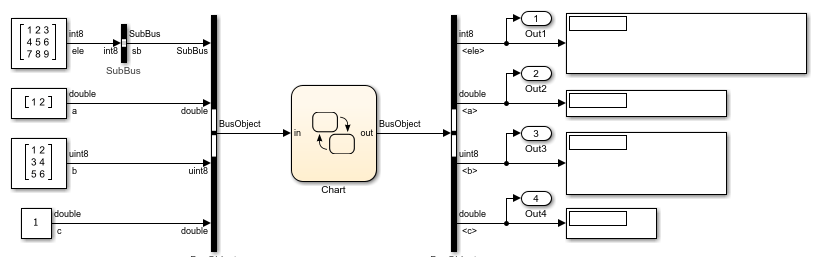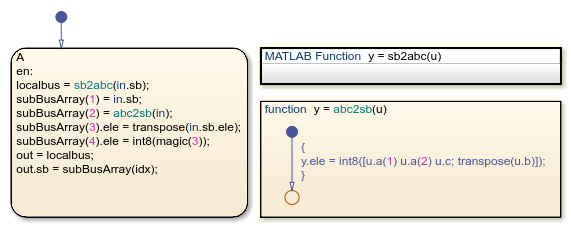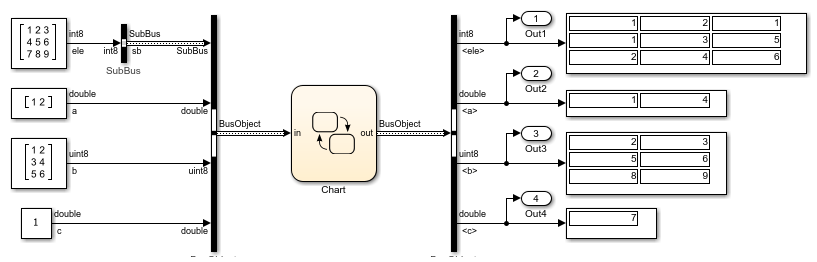# Index and Assign Values to Stateflow Structures

This example shows how to access and modify the contents of a Stateflow® structure. Stateflow structures enable you to bundle data of different sizes and types together into a single `Simulink.Bus (Simulink)` object. Using dot notation and numeric indices, you can access and modify the contents of a Stateflow structure. For more information, see Access Bus Signals Through Stateflow Structures

### Index Substructures and Fields

To index substructures and fields of Stateflow structures, use dot notation. The first part of a name identifies the parent object. Subsequent parts identify the children along a hierarchical path. When the parent is a structure, its children are individual fields or fields that contain other structures (also called substructures). The names of the fields of a Stateflow structure match the names of the elements of the `Simulink.Bus` object that defines the structure.

For example, the chart in this model contains an input structure (`in`), an output structure (`out`), and two local structures (`localbus` and `subbus`).

• The chart defines the input structure `in`, the output structure `out`, and the local structure `localbus` by using the `Simulink.Bus` object `BusObject`. These structures have four fields: `sb`, `a`, `b`, and `c`. The field `sb` is a substructure defined from the `Simulink.Bus` object `SubBus`.

• The chart defines the local structure `subbus` by using the `Simulink.Bus` object `SubBus`. This structure has one field called `ele`.This list illustrates how the Stateflow chart resolves symbols in dot notation for indexing the fields of these structures:

• `in.c` — Field `c` of input structure `in`.

• `out.sb` — Substructure `sb` of output structure `out`.

• `in.a(1)` — First value of the vector field `a` of input structure `in`.

• `subbus.ele(2,2)` — Value in the second row, second column of field `ele` of local structure `subbus`.

• `in.sb.ele(3,4)` — Value in the third row, fourth column of field `ele` of substructure `in.sb`.

### Assign Values to Structures and Fields

You can assign values to any Stateflow structure, substructure, or a field of a structure with a scope different from `Input`.

• To assign one structure to another structure, define both structures from the same `Simulink.Bus` object in the base workspace.

• To assign one structure to a substructure of a different structure (or the other way around), define the structure and substructure from the same `Simulink.Bus` object.

• To assign a field of one structure to a field of another structure, the fields must have the same type and size. You can define the Stateflow structures from different `Simulink.Bus` objects.

This list presents valid and invalid structure assignments based on the structure specifications for this example:

• `in = localbus;` — Invalid. You cannot write to input structures.

• `out = localbus;` — Valid. Both `out` and `localbus` are defined from the same `Simulink.Bus` object `BusObject`.

• `subbus = in;` — Invalid. The structures `subbus` and `in` are defined from different `Simulink.Bus` objects.

• `subbus = in.sb;` — Valid. The structure `subbus` and the substructure `in.sb` are defined from the same `Simulink.Bus` object `SubBus`.

• `in.sb = subbus;` — Invalid. You cannot write to substructures of input structures.

• `out.sb = subbus;` — Valid. The substructure `out.sb` and the structure `subbus` are defined from the same `Simulink.Bus` object `SubBus`.

• `in.c = out.c;` — Invalid. You cannot write to fields of input structures.

• `out.sb.ele = subbus.ele;` — Valid. The field `out.sb.ele` has the same type and size as the field `subbus.ele` (3-by-3 matrices of type `int8`).

• `localbus.a(1) = in.c;` — Valid. The value `localbus.a(1)` has the same type and size as the field `in.c` (scalars of type `double`).

For instance, in the entry action for state `A`, the chart calls the functions `abc2sb` and `sb2abc` and stores the results in the local structures `localbus` and `subbus`. Then, the chart writes to the output structure `out` by combining the values of the local structures `localbus` and `subbus`.The graphical function `abc2sb` takes a structure of type `BusObject` and returns a structure of type `SubBus`. The function combines the values of the fields `a`, `b`, and `c` from its input and rearranges them in a 3-by-3 matrix of type `int8`. Then, the function stores this matrix as the field `ele` of the output structure.The MATLAB® function `sb2abc` takes a structure of type `SubBus` and returns a structure of type `BusObject`. The function decomposes the value of the field `ele` from its input into three components: a vector, a 3-by-2 matrix, and a scalar. The function stores these components in a local MATLAB `struct` `Y` that has the same structure as the `Simulink.Bus` object `BusObject`. Then, the function assigns the value of the MATLAB `struct` `Y` to the output structure `y`.

```function y = sb2abc(u) ```
```% extract data from input structure ```
```E = int8(magic(3)); A = double(u.ele(1:2,1)); B = uint8(u.ele(:,2:3)); C = double(u.ele(3,1)); ```
```% create local structure ```
```X = struct('ele',E); Y = struct('sb',X,'a',A,'b',B,'c',C); ```
```% assign value to output structure ```
```y = Y; end ```

### Run the Simulation

When you simulate the example, the chart uses the values of the field `sb` of the input bus to populate the fields `a`, `b`, `c` of the output bus. The chart also uses the values of the fields `a`, `b`, `c` of the input bus to populate the field `sb` of the output bus.